Question

# 12. The director of admissions at the University of Maryland, University College is concerned about the...

12. The director of admissions at the University of Maryland, University College is concerned about the high cost of textbooks for the students each semester. A sample of 25 students enrolled in the university indicates that x(bar)=\$315.40 and s=\$43.20.

Using a significance level of 0.1, what are the boundaries of the confidence interval?

a. 302.438 - 326.184

b. 300.618 – 330.182

c. 297.485 – 327.820

d. 282.871 – 338.475

13. The director of admissions at the University of Maryland, University College is concerned about the high cost of textbooks for the students each semester. A sample of 25 students enrolled in the university indicates that x(bar)=\$315.40 and s=\$43.20.

A mean that is not in a confidence interval is rejected by the confidence interval, and we say the evidence against the mean is significant. At the 0.10 level of significance, is there evidence against mean \$300?

a. No, because 300 is below the lower limit of the confidence interval.

b. Yes, because 300 is below the lower limit of the confidence interval.

c. No, because 300 is in the confidence interval.

d. Yes, because 300 is in the confidence interval.

14. The director of admissions at the University of Maryland, University College is concerned about the high cost of textbooks for the students each semester. A sample of 25 students enrolled in the university indicates that x(bar)=\$315.40 and s=\$75. (Note the sample standard deviation is different from the first part).

A mean that is in a confidence interval is not rejected by the confidence interval, and we say the evidence against the mean is not significant. At the 0.05 level of significance, is there evidence against mean \$300?

a. No, because 300 is below the lower limit of the confidence interval.

b. Yes, because 300 is below the lower limit of the confidence interval

c. No, because 300 is in the confidence interval

d. Yes, because 300 is in the confidence interval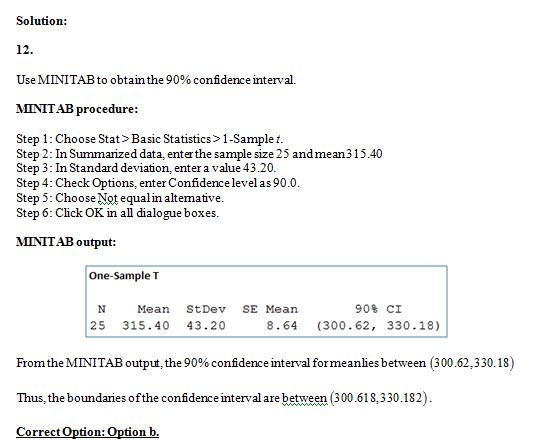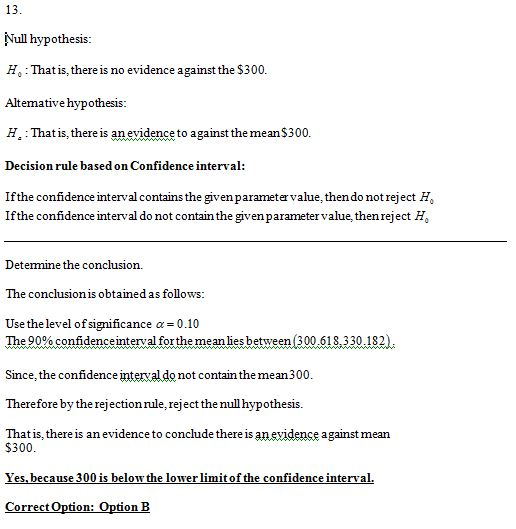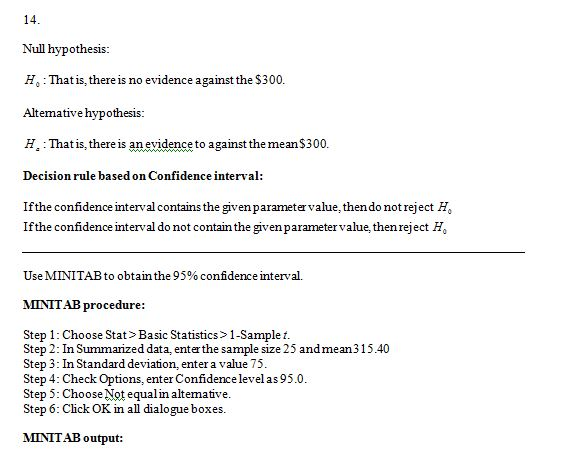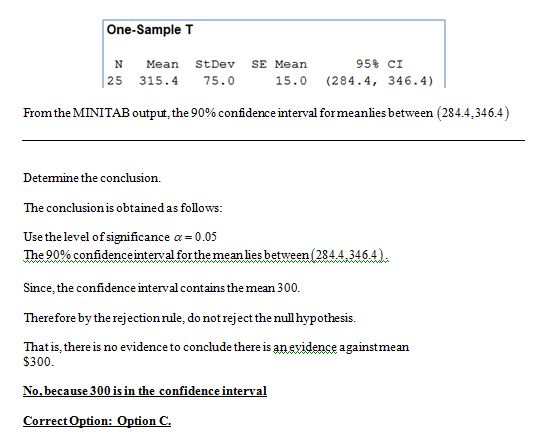#### Earn Coins

Coins can be redeemed for fabulous gifts.

Similar Homework Help Questions
• ### A college admissions director wishes to estimate the mean age of all students currently enrolled. In...

A college admissions director wishes to estimate the mean age of all students currently enrolled. In a random sample of 16 students, the mean age is found to be 21.8 years. From past studies, the ages of enrolled students are normally distributed with a standard deviation of 10.1 years. Construct a 90% confidence interval for the mean age of all students currently enrolled. 1. The critical value: 2. The standard deviation of the sample mean: 3. The margin of error:...

• ### A college admissions director wishes to estimate the mean age of all students currently enrolled. In...

A college admissions director wishes to estimate the mean age of all students currently enrolled. In a random sample of 24 students, the mean age is found to be 23.1 years. From past studies, the ages of enrolled students are normally distributed with a standard deviation of 10.6 years. Construct a 90% confidence interval for the mean age of all students currently enrolled. 1. The critical value: 2. The standard deviation of the sample mean: 3. The margin of error:...

• ### A college admissions director wishes to estimate the mean age of all students currently enrolled. In...

A college admissions director wishes to estimate the mean age of all students currently enrolled. In a random sample of 16 students, the mean age is found to be 21.8 years. From past studies, the ages of enrolled students are normally distributed with a standard deviation of 10.1 years. Construct a 90% confidence interval for the mean age of all students currently enrolled. 1. The critical value: 2. The standard deviation of the sample mean: 3. The margin of error

• ### During an orientation event at a large university, incoming students are advised about the cost of...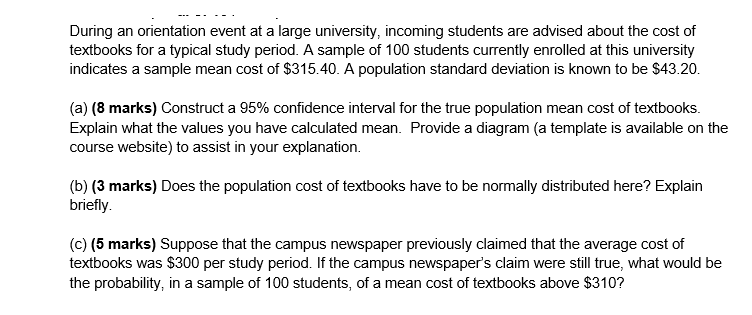During an orientation event at a large university, incoming students are advised about the cost of textbooks for a typical study period. A sample of 100 students currently enrolled at this university indicates a sample mean cost of \$315.40. A population standard deviation is known to be \$43.20. (a) (8 marks) Construct a 95% confidence interval for the true population mean cost of textbooks. Explain what the values you have calculated mean. Provide a diagram (a template is available on...

• ### An admissions director wants to estimate the mean age of all students enrolled at a college....

An admissions director wants to estimate the mean age of all students enrolled at a college. The estimate must be within 1.7 years of the population mean. Assume the population of ages is normally distributed. ​(a) Determine the minimum sample size required to construct a 90% confidence interval for the population mean. Assume the population standard deviation is 1.8 years. ​(b) The sample mean is 20 years of age. Using the minimum sample size with a 90% level of​ confidence,...

• ### An admissions director wants to estimate the mean age of all students enrolled at a college....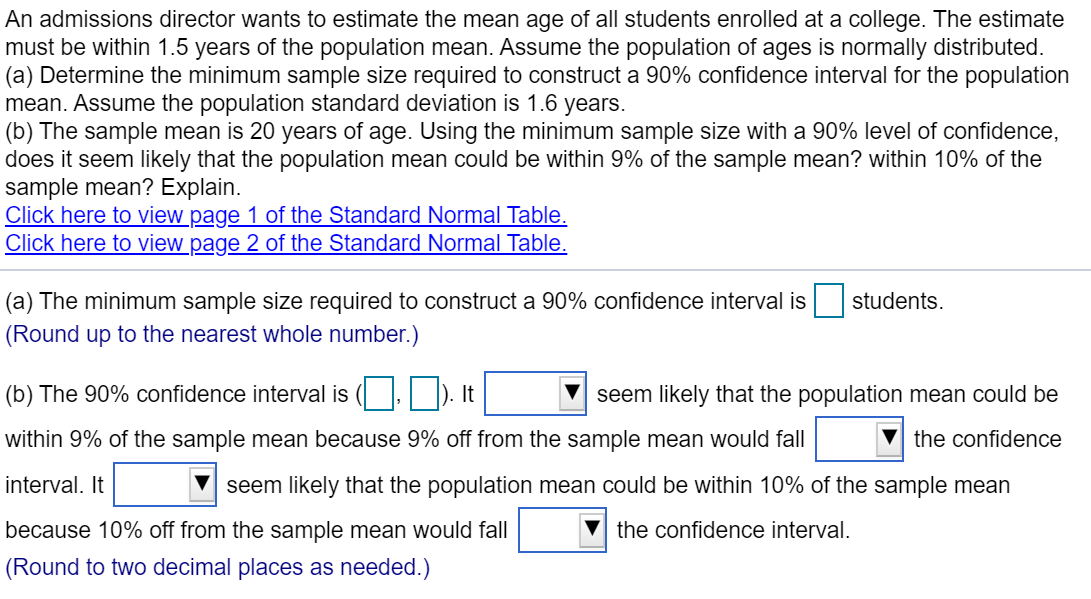An admissions director wants to estimate the mean age of all students enrolled at a college. The estimate must be within 1.5 years of the population mean. Assume the population of ages is normally distributed. (a) Determine the minimum sample size required to construct a 90% confidence interval for the population mean. Assume the population standard deviation is 1.6 years. (b) The sample mean is 20 years of age. Using the minimum sample size with a 90% level of confidence,...

• ### An admissions director wants to estimate the mean age of all students enrolled at a college....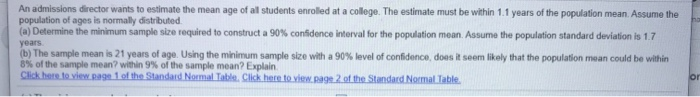An admissions director wants to estimate the mean age of all students enrolled at a college. The estimate must be within 1.1 years of the population mean. Assume the population of ages is normally distributed (a) Determine the minimum sample size required to construct a 90% confidence interval for the population mean. Assume the population standard deviation is 1.7 years (b) The sample mean is 21 years of age. Using the minimum sample size with a 90% level of confidence,...

• ### A public relations manager for the Cincinnati Reds wants to determine the percentage of local college...

A public relations manager for the Cincinnati Reds wants to determine the percentage of local college students who attended a Reds baseball game at Great American Ballpark during the 2016 season. A sample of 648 students who are enrolled at a college or university within 25 miles of the Reds ballpark was asked whether or not they attended at least one Cincinnati Reds game during the 2016 season. Out of the 648 students asked, 246 had attended a game during...

• ### A college’s admissions guide state that students spend approximately \$300 for textbooks each semester. A random...

A college’s admissions guide state that students spend approximately \$300 for textbooks each semester. A random sample of 31 college students finds that the sample mean for the amount spent on textbooks is \$365. Assume that the standard deviation for the population is \$75. Test at the a = .02 level to determine if students spend significantly more than the amount stated in the admission’s guide.

• ### A publishing company claims that in fall 2019, the average price of college textbooks for a...

A publishing company claims that in fall 2019, the average price of college textbooks for a single semester is \$385. Suppose you decide to collect data from a random sample of students to assess whether the publisher's claim is reasonable, and you find that in a random sample of 22 college students, the mean price of textbooks for the fall 2019 semester was \$433.50 with a standard deviation of \$86.92.   At the 0.01 significance level, is there sufficient evidence to...